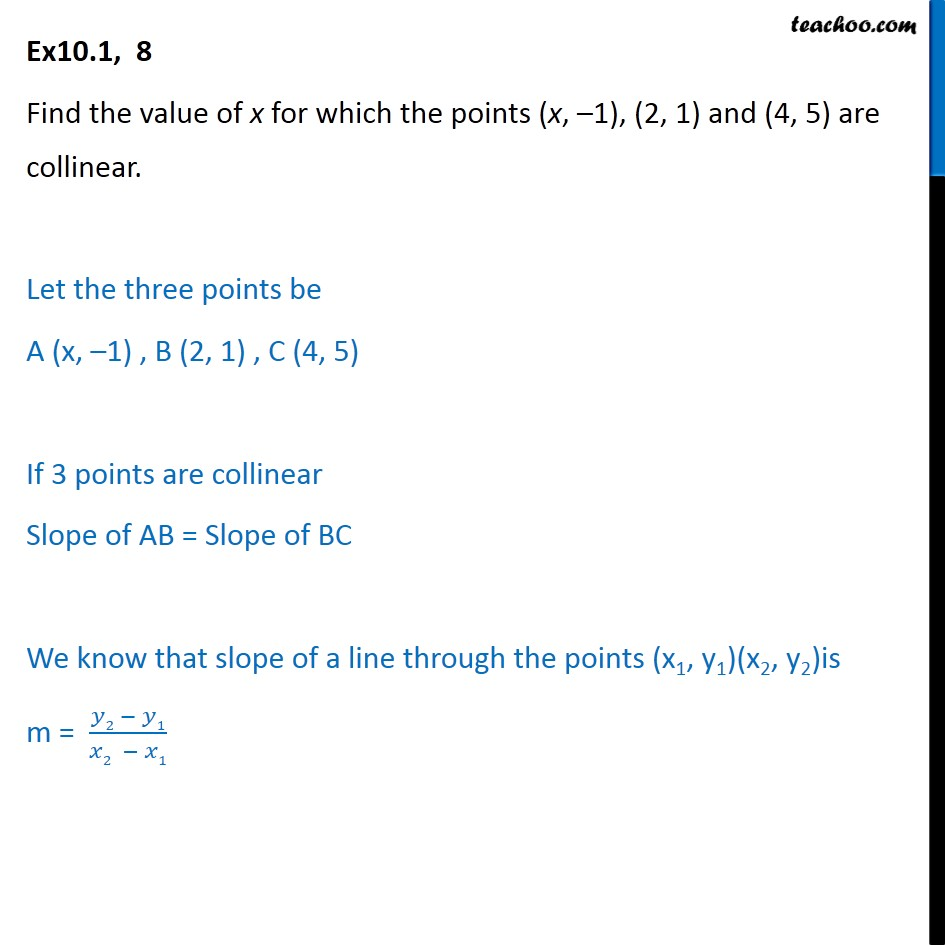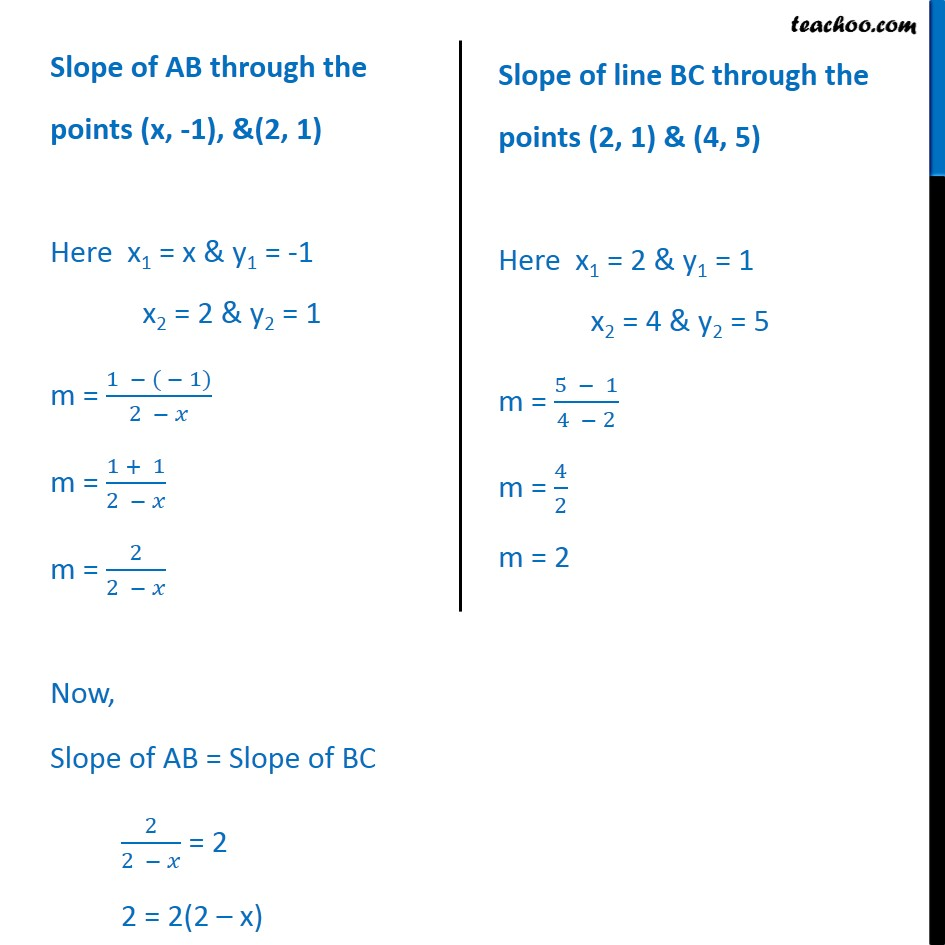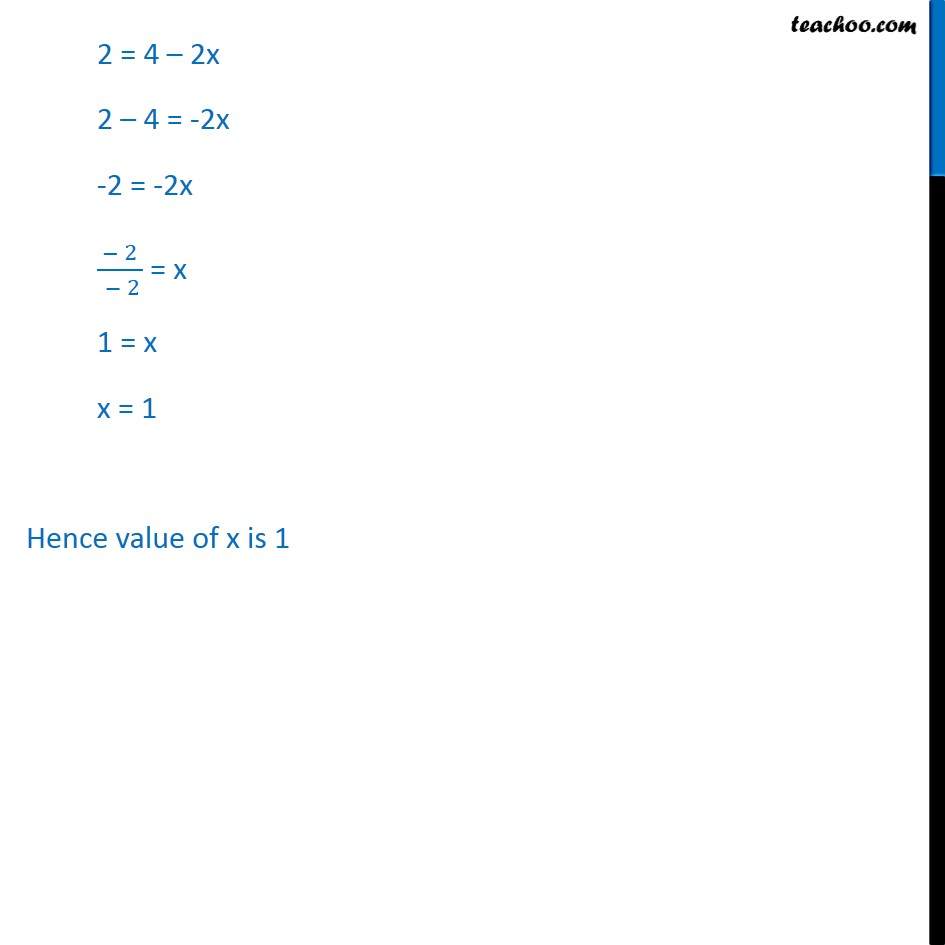Ex 9.1

Chapter 9 Class 11 Straight Lines
Serial order wiseLearn in your speed, with individual attention - Teachoo Maths 1-on-1 Class

### Transcript

Ex10.1, 8 Find the value of x for which the points (x, –1), (2, 1) and (4, 5) are collinear. Let the three points be A (x, –1) , B (2, 1) , C (4, 5) If 3 points are collinear Slope of AB = Slope of BC We know that slope of a line through the points (x1, y1)(x2, y2)is m = (𝑦2 − 𝑦1)/(𝑥2 − 𝑥1) 2 = 4 – 2x 2 – 4 = -2x -2 = -2x ( − 2 )/( − 2) = x 1 = x x = 1 Hence value of x is 1